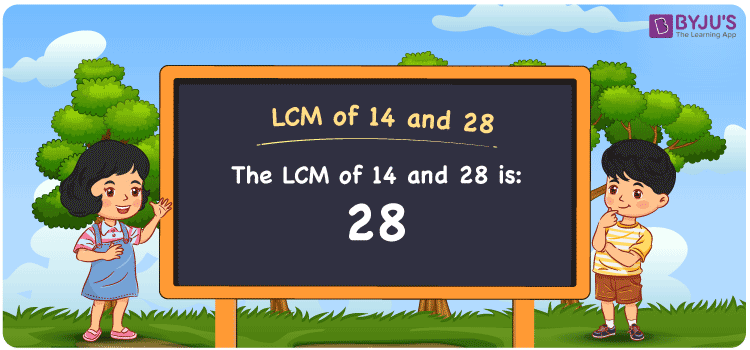Checkout JEE MAINS 2022 Question Paper Analysis : Checkout JEE MAINS 2022 Question Paper Analysis :

# LCM of 14 and 28

LCM of 14 and 28 is 28. The smallest number which is evenly divisible among the multiples of the 14 and 28 indicates the LCM. By glancing at the common multiples, the least common multiple of 14 and 28 can be determined. (14, 28, 42, 56, 70, 84, ….) and (28, 56, 84, 112, 140,….) are the multiples of 14 and 28. You can find the LCM value using the prime factorisation method, division method and listing the multiples.

## What is LCM of 14 and 28?

The answer to this question is 28. The LCM of 14 and 28 using various methods is shown in this article for your reference. The LCM of two non-zero integers, 14 and 28, is the smallest positive integer 28 which is divisible by both 14 and 28 with no remainder.## How to Find LCM of 14 and 28?

LCM of 14 and 28 can be found using three methods:

• Prime Factorisation
• Division method
• Listing the multiples

### LCM of 14 and 28 Using Prime Factorisation Method

The prime factorisation of 14 and 28, respectively, is given by:

14 = 2 x 7 = 2¹ x 7¹

28 = 2 x 2 x 7 = 2² x 7¹

LCM (14, 28) = 28

### LCM of 14 and 28 Using Division Method

We’ll divide the numbers (14, 28) by their prime factors to get the LCM of 14 and 28 using the division method (preferably common). The LCM of 14 and 28 is calculated by multiplying these divisors.

 2 14 28 2 7 14 7 7 7 x 1 1

No further division can be done.

Hence, LCM (14, 28) = 28

### LCM of 14 and 28 Using Listing the Multiples

To calculate the LCM of 14 and 28 by listing out the common multiples, list the multiples as shown below

 Multiples of 14 Multiples of 28 14 28 28 56 42 84 56 112 70 140 84 168

LCM (14, 28) = 28

## Video Lesson on Applications of LCM## LCM of 14 and 28 Solved Examples

If the GCD is 14 and the product of two numbers is 392, find the LCM.

Solution:

It is given that

Product of two numbers = 392

GCD = 14

We know that

LCM x GCD = Product of two numbers

LCM = Product/GCD

LCM = 392/14

LCM = 28

Hence, the LCM is 28.

## Frequently Asked Questions on LCM of 14 and 28

### What is the LCM of 14 and 28?

The LCM of 14 and 28 is 28.

The smallest multiple exactly divisible by 14 and 28 should be found.

Multiples of 14 = 14, 28, 42, 56, 70, 84, …

Multiples of 28 = 28, 56, 84, 112, 140, 168, ….

The smallest common multiple exactly divisible by 14 and 28 is 28.

### List the methods which can be used to get the LCM value of 14 and 28.

The methods which can be used to get the LCM of 14 and 28 are the Prime Factorization Method, Division Method and Listing multiples.

### What is the GCF if the LCM of 14 and 28 is 28?

LCM x GCF = 14 x 28

Given

LCM of 14 and 28 = 28

28 x GCF = 392

GCF = 392/28 = 14